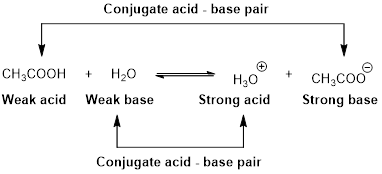Chapter 16, Problem 22PS

Chapter
Section
Textbook Problem

Which of the following compounds or ion has the strongest conjugate acid? Briefly explain your choice. (a) CN− (b) NH3− (c) SO42−

Interpretation Introduction

Interpretation:

The strongest conjugate acid has to be identified from the given ions or compounds.

Concept introduction:

An equilibrium constant (K) is the ratio of concentration of products and reactants raised to appropriate stoichiometric coefficient at equlibrium.

For the general acid HA,

HA(aq)+H2O(l)H3O+(aq)+A(aq)

The relative strength of an acid and base in water can be also expressed quantitatively with an equilibrium constant as follows:

Ka=[H3O+][A][HA]

An equilibrium constant (K) with subscript a indicate that it is an equilibrium constant of an acid in water.

For the general base B,

B(aq)+H2O(l)BH(aq)+OH(aq)

The relative strength of an acid and base in water can be also expressed quantitatively with an equilibrium constant as follows:

Kb=[BH][OH][B]

An equilibrium constant (K) with subscript b indicate that it is an equilibrium constant of base in water.

Acid dissociation constant reflects the equilibrium that exists for an acid solution.

Base dissociation constant Kb reflects the strength of base. The higher the value of Kb stronger the base.

Bronsted –Lowry conjugated acid-Base pairs:

When an acid is dissolved in water, the acid (HA) donates a proton to water to form a new acid (conjugated acid) and a new base (conjugated base).The pair of an Acid –Base differs by a proton called conjugated Acid-Base pair.

Explanation

Reason for the correct statement

For the general acid HA,

HA(aq)+H2O(l)H3O+(aq)+A(aq)

Ka=[H3O+][A][HA]

A large value of Ka indicates that the ionization product is strongly favoured because the product [H3O+][A] is more than the equilibrium concentration of the strong acid [HA].

A conjugate acid-base pair contains two compounds that differ only by a hydrogen ion and a charge of +1. Thus, HA and A are conjugate acid-base pair. In this pair, A is a conjugate base of an acid HA.

The weaker the acid, the stronger its conjugate base and vice-versa. That is, the smaller the value of Ka, the larger the value of Kb.

1. a) CN-

The ionization reaction is as follows.

HCN H+ + CN-   Kb= 2.5×10-5

1. b) NH3

The ionization reaction is as follows.

NH4++ H2NH3 + H3O+     Kb= 1.8×10-5

1. c) SO42-

The ionization reaction is as follows

Still sussing out bartleby?

Check out a sample textbook solution.

See a sample solution

The Solution to Your Study Problems

Bartleby provides explanations to thousands of textbook problems written by our experts, many with advanced degrees!

Get Started

8.35 What is an instantancous dipole?

Chemistry for Engineering Students

Why do distant stars look redder than their spectral types suggest?

Horizons: Exploring the Universe (MindTap Course List)

What is the basis for the name citric acid cycle?

General, Organic, and Biological Chemistry